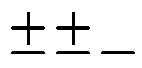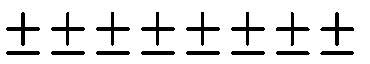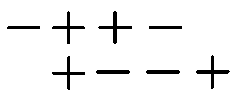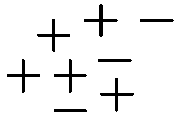### Home > MC1 > Chapter 2 > Lesson 2.2.2 > Problem2-66

2-66.

Write an expression to represent each of the following sketches. Then give the value of each sketch.

1.Look for pairs of positive and negative integers that will add to zero.

There are two pairs that add to zero and one non-paired negative integer.

Write an expression representing the integers that added to zero, plus the remaining integers.

$0+0+(−1)$

One solution to this problem is $0+0+(−1)=−1$.
You can also write this as $2+(−3)=−1$.

1.To solve this problem, follow the same steps as in part (a).

One solution to this problem is $0+0+0+0+0+0+0+0=0$.
Another is $8+(−8)=0$.

1.How many $+$'s are there? How many $−$'s? How many zeros?

1.Follow the same steps as in part (a).